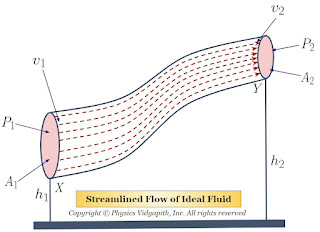## Geothermal Energy

Geothermal energy is a form of renewable energy that is generated by the natural heat of the Earth's core. This heat is constantly produced by the decay of radioactive isotopes deep within the Earth's crust, and it is estimated that the Earth's core could generate up to 45 terawatts of power, which is more than the world's total energy consumption.

Geothermal energy is typically extracted by drilling deep wells into the Earth's crust to access hot water and steam. The heat is then used to generate electricity or provide heating and cooling for buildings. There are three main types of geothermal energy systems: dry steam, flash steam, and binary cycle.

In dry steam systems, steam is directly extracted from the ground and used to power turbines, which generate electricity. This method is used in areas where the temperature of the steam is high enough to drive the turbines without the need for additional heating.

In flash steam systems, hot water is pumped up from the ground and then sprayed into a flash tank at a lower pressure. This causes the water to vaporize into steam, which is then used to power turbines. This method is commonly used in areas where the temperature of the water is high, but not high enough to produce steam naturally.

In binary cycle systems, hot water is pumped up from the ground and passed through a heat exchanger, where it is used to heat a secondary fluid with a lower boiling point. The secondary fluid vaporizes, which drives a turbine and generates electricity. This method is used in areas where the temperature of the water is lower and cannot produce steam directly.

One of the advantages of geothermal energy is that it is a renewable and sustainable source of energy, as the heat from the Earth's core is constantly being produced. It is also a reliable source of energy, as it is not dependent on weather conditions, such as wind or sunlight, to generate power.

Geothermal energy is also a relatively clean source of energy, as it produces very little greenhouse gas emissions. However, there are some environmental concerns associated with geothermal energy, such as the release of gases, such as hydrogen sulfide and carbon dioxide, during the drilling and production process.

Another disadvantage of geothermal energy is that it is not available in all areas, as it requires certain geological conditions, such as a high temperature gradient and permeable rock formations, to be present. Additionally, the initial costs of building geothermal power plants can be high, as they require significant investments in drilling and infrastructure.

Despite these challenges, geothermal energy has the potential to play an important role in the transition to a more sustainable and low-carbon energy system. With continued research and development, it is possible that geothermal energy could become a more widely available and cost-effective source of renewable energy in the future.

## Popular Posts

### Bernoulli's Theorem and Derivation of Bernoulli's EquationStatement of Bernoulli's Theorem: When an ideal fluid (i.e incompressible and non-viscous Liquid or Gas) flows in streamlined motion from one place to another, then the total energy per unit volume (i.e Pressure energy + Kinetic Energy + Potential Energy) at each and every of its path is constant. $P+\frac{1}{2}\rho v^{2} + \rho gh= constant$ Derivation of Bernoulli's Theorem Equation: Let us consider that an incompressible and non-viscous liquid is flowing in streamlined motion through a tube $XY$ of the non-uniform cross-section. Now Consider: The Area of cross-section $X$ = $A_{1}$ The Area of cross-section $Y$ = $A_{2}$ The velocity per second (i.e. equal to distance) of fluid at cross-section $X$ = $v_{1}$ The velocity per second (i.e. equal to distance) of fluid at cross-section $Y$ = $v_{2}$ The Pressure of fluid at cross-section $X$ = $P_{1}$ The Pressure of fluid at cross-section $Y$ = $P_{2}$ The height of cross-section $X$ from